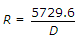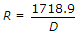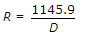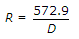# Civil Engineering - UPSC Civil Service Exam Questions

36.

For a simple circular curve which one of the following gives the correct relation between the radius R and degree of curve D, for 20 m arc length ?

 A.B.C.D.Explanation:

No answer description available for this question. Let us discuss.

37.

A car negotiates a curve on a slippery road at a constant speed of 10 m/s. If the coefficient of friction is 0.5, what is the minimum radius of the arc in which the car can turn ?

 A. 20 m B. 10 m C. 5 m D. 4 m

Explanation:

No answer description available for this question. Let us discuss.

38.

Grid lines on a mapsheet can be plotted mechanically by means of a

 A. rotometer B. pantograph C. coordinatograph D. stereometer

Explanation:

No answer description available for this question. Let us discuss.

39.

A municipal sewage has BOD5 of 200 mg/l. It is proposed to treat it and dispose off into a marine environment. For what minimum efficiency should the sewage treatment plant be designed ?

 A. 85% B. 60% C. 50% D. 33.67%

Explanation:

No answer description available for this question. Let us discuss.

40.

As the state of strain of an element of dense sand changes from plain strain to trivial condition, the effective angle of internal friction :

 A. increases B. decreases C. remains constant D. first increases and then remains constant